International Journal of Genetics and Genomics
Volume 4, Issue 3, June 2016, Pages: 24-30

The Method of a Gene Sequence Alignment BWT Index Based on Hadoop

Nan Li1, Jing Gao1, *, Bailong Feng2

1College of Computer and Information Engineering, Inner Mongolia Agricultural University, Hohhot, Inner Mongolia, China

2East Inner Mongolia Electric Power Company Limited, Hohhot, Inner Mongolia, China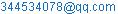(Nan Li)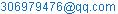(Bailong Feng)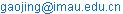(Jing Gao)

*Corresponding author

Nan Li, Jing Gao, Bailong Feng. The Method of a Gene Sequence Alignment BWT Index Based on Hadoop. International Journal of Genetics and Genomics. Vol. 4, No. 3, 2016, pp. 24-30. doi: 10.11648/j.ijgg.20160403.13

Received: February 26, 2016; Accepted: June 17, 2016; Published: July 12, 2016

Abstract: Gene sequence alignment, used to recognize the homology and variability in different species, is an important part of Bioinformatics. Creating indexes is a crucial step of gene sequence alignment algorithm. Usual algorithms of creating indexes are divided into two types. The first is algorithm based on hash table, while another is based on suffix tree or suffix array, among which BWT (Burrows-Wheeler Transform) index is a significant index structure. Currently, BWT index needs several hours’ serial computing in building a large genome sequence (such as human genome sequence). A parallel computing method based on Hadoop is presented is this paper to build suffix array and BWT index.Map Reduce is adopted as a type of data processing function, cutting suffix array into block, which will be handled separately. Ultimately, totally ordered suffix array and BWT index are output, reducing the time in building index. Meanwhile, verifying the effectiveness of the algorithm by experiments.

Keywords: Gene Sequence, BWT Index, Suffix Array, Hadoop

1. Introduction

As the changing of an organism's genome length, from an simple bacterial organism's millions of nucleotides to the largest known three billion nucleotides in the genome, the search and analysis methods of genome is gradually improved. They rely on the index structure, and is able to match and query quickly, at the same time canning each nucleotide individuals with no loss of sensitivity. And except for a few short sequences, a lot of sequence alignment algorithms require preprocessing, calculating the index structure of the sequences that need to be compared in advance. So the method researched in this paper is of great help to the index structure of the sequence alignment algorithm.

The common index construction algorithm is roughly divided into two kinds , one is based on the hash table, and BLAST  is a typical representative based on the hash table algorithm. Another kind is based on suffix tree and suffix array, which include the suffix tree [3,4] , enhanced suffix array, FM – Index . In this paper, we study the second algorithm.

BWT index is an important index structure based on suffix array algorithm. BWT index’s operand is data block, and sort character string based on suffix array. At present, the software using this method include Bowtie , such as, BWA , BWT – SW , SOAP2, etc.

BWT index needs a few hours’ computing at stand-alone calculation. If there is not enough memory to save the entire suffix array, it will be more complicated. Current parallel technology of suffix array structure includes DC3 algorithm based on MPI, which mainly divides suffixes into two parts, and then sorts the first part of the suffix. According to the result of the first part, sort the second part, and then combine the two parts to get the final result. This paper put forward a parallel algorithm based on Map Reduce computing framework to build gene sequences’ suffix array and BWT index, using Hadoop open source platform, named the BWH algorithm. The algorithm use Map Reduce computing framework’ data processing function, cutting the suffix array into multiple data blocks, and calculate them independently. In order to avoid the load balancing of node, BWH algorithm allocates tasks averagely, according to the total number of Map tasks and Reduce tasks, letting each node process core issues relatively evenly. By comparing BWH algorithm and suffix sorting algorithm--the basic algorithm based on BWT, BWH algorithm reduced the amount of time to establish BWT index obviously, and improve the efficiency of the operation

In this paper, the basic structure is as follows: The first section mainly describes the background information and the significance of research; The second section is the overview of basic knowledge of theory and related technology about BWT index; The third section introduces the implementation process of BWH algorithms pecifically; The fourth section describes the simulation of experimental results and analysis; The fifth section, as a conclusion, summarize the work done by now and the places that can be further improve.

2. Basic Theory and Related Technology Overview

2.1. The Basic Principle of BWT Index and Suffix Sorting Algorithm

Building process of BWT index is as follows:

The first step, each base (A, T, C, G) in the sequence move back one place in turn. Every move of base can produce a new string. Assume that sequence S is ATTCGA, whose length is 6. The sequence will move 5 times, at the same time produce five new sequences, composing BWT matrix T, as is shown in figure 1.

The second step, for the matrix T, sort each line in alphabetical order to obtain a new BWT matrix T’. The number of rows in the original matrix (matrix T) that the ith line of matrix T’ corresponds to is the array S[i]. The bases in the last row of the matrix T' after the rotation is the array B [i]. The sequence can be restored according to the two arrays.

The third step, to build an array of Oc(A) to display the number of line of the first base line of A in the matrix T’. Build array Occ (1, G) to display the last column in the matrix T'.G in line 1 is in which column.

It's worth noting that the first character of BWT is always the last word before \$ character . In addition, the ith line in the BWT string is the prior character of the ith suffix in the suffix array. The suffix array field records the starting position of each suffix of the original string.

The matching point is quickly found according to the the two arrays Oc (r) and Occ (BWT [r], r) as the index. One of the core operation of building an index is constructing BWT list. BWT string along with Oc (r) and Occ (BWT [r], r) constitutes the BWT index .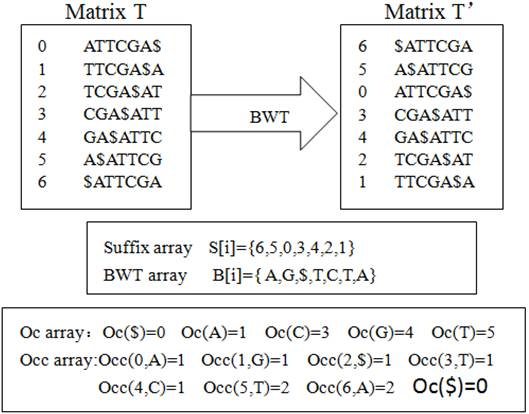Figure 1. Schematic siagram of suffix index based on BWT.

Sorting the suffix S1, S2,...Si of a sequence S. Starting from the first suffix S1 to the last suffix Si sort in dictionary order. When all the suffixes are sorted, the collection is called suffix list T. Replace all of the suffixes in the suffix list T with the prior character in S, forming a BWT string that is corresponding to sequence S. This algorithm is called suffix sorting algorithm .

The founder of Hadoop is doug Cutting, the originator of Apache Lucene [13, 14]. Hadoop platform, an open-source software framework, is developed by the Apache software foundation, and is able to process mass data by distributed processing . This article mainly use Map Reduce computing framework of Hadoop platform when indexing (shown in figure 2).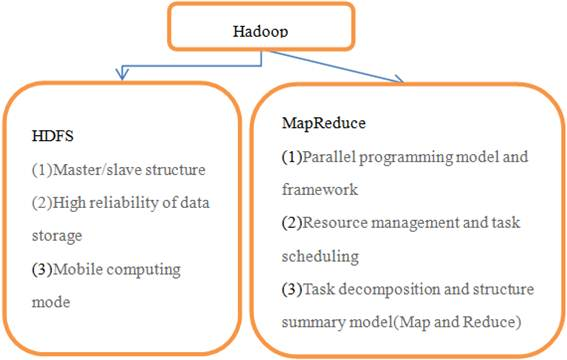Figure 2. Major component of Hadoop.

The Map Reduce is mainly divided into two parts, the Map and Reduce. First, cut the input data into several separate data slots according to the size of data block and the size of data. These pieces of data will be divided into different Map. After the processing in Map stage, generate intermediate data like <key, value>. Transmit data to Reduce function after shuffling and sorting process. The Reduce function receives the input in the form of<key, (the list of value)>, then deals with the value collection and generate the final result . The data flow diagram of Map Reduce is shown in figure 3.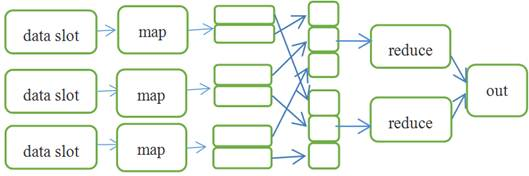Figure 3. Data flow diagram of MapReduce.

3. Bwt the Index Method of Establishing Based on the Ratio of Gene Sequences Hadoop Platform

This paper presents an algorithm for constructing BWT index which was called BWH. Using Map Reduce to get the method of suffix array and BWT string is to use the Map Reduce to divide the tasks into different groups. Then the data distributed in each group will be calculated in different Reduce. Through the shuffle stage the algorithm can get new keys for sorting and summarizing. In this paper, according to certain rules, the original gene sequence is devided into several short sequences. At each node, the short sequence is calculated and sorted.

The realization of the algorithm is as follows:

Definition 1: given a set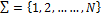, the elements in this set are the value of BWH. N is the lengh of the sequence. According to the characteristics of the BWH algorithm, assuming the value of the constant α is 18(the value of α is the length of the key in BWH algorithm’s Map phase), traverse the key value in each of the Reduce. Let i(1<=i<=N) be an element of the set, and according to the i+α, determine the corresponding new key value.

In this paper, the segmentation rules are defined as follows:

Definition 2: assuming the gene sequence as S, move the first base of the S to the end, get S1. Use α bases in the end of S1 to form the key1. At the same time, add a separator at the end of S1, performing the split process to form a Map task. Remove the separator at the end of S1, and move the first base of the S1 to the end of S1, then the gene sequence changed from S1 to S2, and add a separator at the end of the S1, and so on.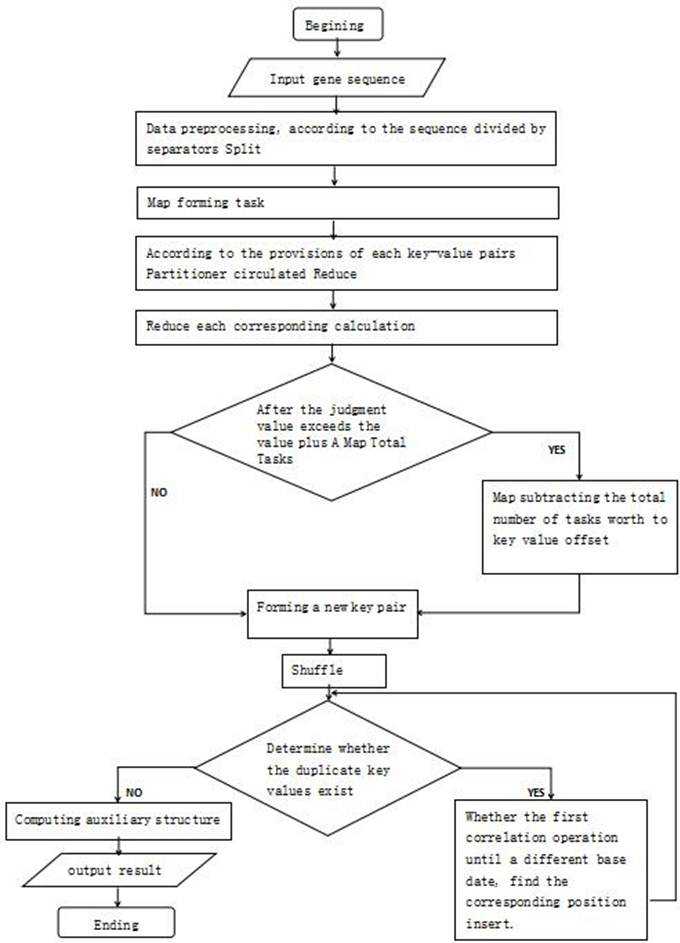Figure 4. Algorithm flow chart of BWH algorithm.

Algorithm is described as follows:

Input: we need to set up an index of gene sequences. BWH algorithm prepare a document for each key of reference string. That is, 1, 2,……N, N is the length of the sort sequence.

Output:

Output the suffix array and BWT index to construct the index of the gene sequence.

The first step, segment data: According to the definition 2, form the independent Map task. The forming process of key is shown in figure 5.

The second step, data distribution: In order to avoid unbalanced load of the task distribution, BWH algorithm assign tasks relatively averagely based on the total number of Map tasks and Reduce tasks. Total number of Map tasks is N, M is the number of Reduce. The tasks assignment make Map tasks do continuous value assignment according to result of N / M.

The third step, calculate: According to the calculation process of definition 1, we can get new key-value pairs.

The fourth step, judgment operation: Analysis the result of (α+value) and the total number of Map tasks, if the former exceeds the total number of Map tasks, the offset of the key will be the total number of Map tasks subtract the value. According to the offset of key to form key-value pairs. Otherwise, form key-value pairs directly.

The fifth step, Shuffle stage: Use default Shuffle stage of Hadoop, sorting all key-value pairs with lexicographic order.

The sixth step, judgment operation: Analysis whether the value of each key are the same. If there is repeated key value, make it plus α, and compare it with the former 2α. If there is still repeated key value, make it plus 2α to compare with former 3α of the key value. Do the same thing until the first different base is found.

The seventh step, termination condition: No duplicate key values and fully sorted.

The eight step, other calculations: Calculate aided structure Oc array and Occ array.

The nine step, output result: Output the suffix arrays of the gene sequences which need to build index and BWT index.

Pseudo-code of the algorithm is as follows:

(1)Mapper process pseudo-code:

Input S

l<--S.lenth

S<--S+"\$"

While  i<l do

begin

c<--string.charAt(0)

S<--S.subString(1,l-1)+c+" "

(startIdx, endIdx)<——split(key," ")

S<--S.trim()

i<--i+1

until i=l

end

}

}

(2)Partitioner process pseudo-code:

Input (key S,value)

If(value<α)  then

do

substr<——S.substr(S.lenth-1-(1+value),S.lenth-1)

else

substr <——S.substr(S.lenth-1-α,S.lenth-1)

return mer(substr)/numReducers+α

(3)Reduce process pseudo-code:

Input(key str,value)

for i (1+reduce(k)*(mer(substr)/numReducers)) to (mer(substr)/numReducers)

for j (reduce(k)*mer(substr)/numReducers) to (mer(substr)/numReducers)

if (valuej-valuei=α) then

do

begin

get(keyj,valuei)

else if(valuei+<mer(substr))

do

get(keyj+α,valuei)

else

do

stringEnd<——S.substr(17-(mer(substr)-valuei),17)

get(stringEnd,valuei)

endFigure 5. Forming process of key.

4. Analysis of Experimental Results

In this paper, Hadoop-0.20.2 is built on the VMware vSphere virtual platform. The computer system is RedHat Linux6.3. There are 15 nodes in this experiment. Conduct related experiments to realize the parallel suffix array and BWT index construction algorithm. The whole experiment is conducted by Java. During the experiment, we successfully built suffix array and BWT index through Map Reduce computing framework of Hadoop. Experimental data are real data downloaded in the NCBI database. 5 sequences of the experiment include a human gene sequences, a mouse gene sequences, a corn gene sequences, a parrot gene sequence and a sheep gene sequence .

Experiment uses vSphere virtual platform, which is made up of some physical machines with EXSI operating system and a physical machine with Vcenter controller. In this paper, experiment uses private IP address. That is class C IP address (192.168.0.0-192.168.255.0).

Basic configurations of software and hardware of physical machine are shown in table 1. In virtual platform, apply 25 virtual machines from 2 EXSI physical machines to build Hadoop platform and share hardware resources. The basic configurations of hardware and software in these virtual machines are shown in table 2.

Table 1. Hardware and software basic configuration of physical machine.

 physical machine Basic hardware configuration Basic software configuration CPU Core number memory hard disk underlying systems main control software Physical machine 1 E5-4620 V2@2.60GHZ 4 512GB 1TB EXSI5.1 vCenter physical machine 2 E5-4620 V2@2.60GHZ 4 512GB 1TB EXSI5.1

Table 2. The hardware and software basic configuration of each node of virtual machine.

 node type IP Address CPU memory disk physical machine OS Hadoop version name node 192.168.62.2 Two 4 cores 24GB 200GB 162 Red-Hat Enterprise Linux6 (64-bit) hadoop-0.20.2 data node 192.168.62.3-12 Two 4 cores 24GB 70GB 171 data node 192.168.62.13-17 Two 4 cores 24GB 70GB 162

In the rotation of BWH algorithm, this article takes the last eighteen bits after each rotation (if not enough, take all out). According to the calculation method, get first eighteen bases and sort them. There are 418 possibilities as far as the first 18 bits are concerned, so the probability of repetition is very small.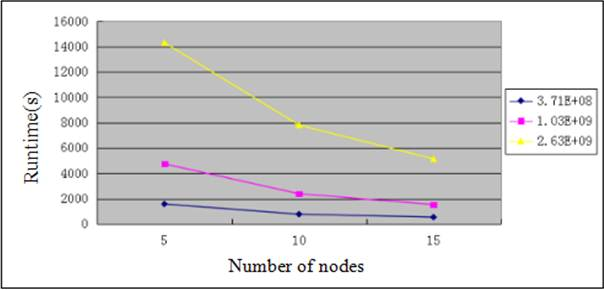Figure 6. The time of BWT index establishment of three sequences under three environments.

Figure 6 shows the running time in three different conditions when constructing BWT index. In the figure, when the sequence length is relatively short, with the increase of the nodes, the time is reducing not obviously. For the larger sequence, algorithm has obvious improvement after using 15 nodes. So using multiple nodes is suitable for larger genomes.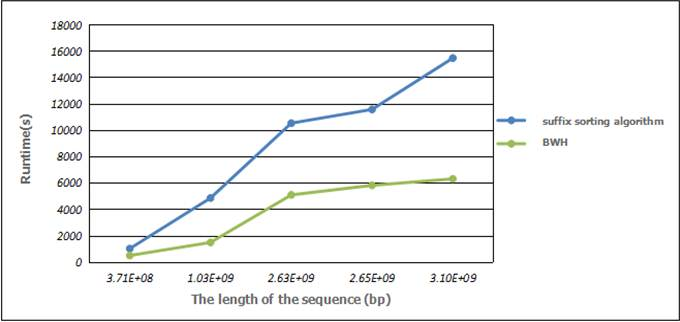Figure 7. The time comparison between suffix sorting algorithm and BWH in building index.

Figure 7 shows the time comparison between suffix sorting algorithm and BWH algorithm in building BWT index. As is shown in figure 7(X-axis is the length of the sequence, Y-axis is running time), with the growth of time, the time of our algorithm in constructing index is significantly less than the suffix sorting algorithm. With the increase of sequences, BWH algorithm can save more time.

5. Conclusion

We build the parallel suffix array and BWT index in the Hadoop 0.20.0. Compared with suffix sorting algorithm, our algorithm has obvious improvement, showing the algorithm is effective. And with the development of cloud computing, Hadoop is more and more applicable. There will be more and more genetic sequence alignment software implemented on Hadoop platform.

Through the above description, calculating BWT index based on Map Reduce, can get the result fast. Although parallel computing BWT index using the Hadoop is the latest currently, we just initially realized BWT index establishment. And there are a lot of unknown regions and a large number of repetitive characters in the genome , that need to be optimized. And this method also has disadvantages. With the increase of nodes, more and more communication time will be wasted when facing large number of data.

Acknowledgment

This work is supported by the Natural Science Foundation of China No.( 61462070), the "ChunHui Plan" project of Educational Department No. (Z2009-1-01062), the research of evaluation technology of security and reliability of cloud computing and the built of testing platform that is a technology plan project of Inner Mongolia No. (20130364).

References

1. ZHAO Ya-Nan Research on BWT index and algorithms in biological sequence alignment [D], University of Science and Technology of China.)2015.
2. MCKENNA A, HANNA M, BANKS E, et al. The Genome Analysis Toolkit: a Map Reduce framework for analyzing next-generation DNA sequencing data. [J]. Genome research, 2010, 20(9):1297-1303.
3. Niek B, Inna D, Lior P. AVID: a global alignment Program. Genome Research [J]. 2003, 13(1): 97–102.
4. Delcher A L, Phillippy A, Carlton J, et al. Fast algorithms for large-scale genome alignment and comparison [J]. Nucleic Acids es, 2002, 30(11): 2478–2483.
5. Kurtz S, Phillippy A L, Smoot M, et al. ersatile and open software for comparing large genomes[J]. Genome Biol, 2004, 5(2): R12.
6. FERRAGINA P, MANZINI G., MAKINEN V, et al. AN Alphabet-Friendly FM-index[C]//APOSTOLICO A, MELUCCI M.11th International
7. LANGMEAD B, TRAPNELL C, POP M, et al. Ultrafast and memory-efficient alignment of short DNA sequence to the human genome [J]. Genome Biology, 2009, 10(3): R25.
8. Li H, Durbin R. Fast and accurate long-read alignment with" Burrow Wheeler" transform [J]. Bioinformatics, 2010, 26(5):589-595.
9. LAMT W, SUNG WK, TAM SL, et al. Compressed indexing and local alignment of DNA [J]. Bioinformatics, 2008, 24(6): 791-797.
10. Burrows M. A block-sorting lossless data compression algorithm [J]. Technical Report Digital Src Research Report, 1994, 57(4): 425.
11. GAO Jing, JIAO Ya ZHANG Wen-Guang Overview of Sequence Alignment for HIGH-throughput Sequencing Data[J] LIFE SCIENCE RESEARCH 2014(05).
12. Li Bing, Long Bing-jie, Liu Yong. A Fast Algorithm for Burrows-Wheeler Transform Using Suffix Sorting [J]. Journal of Electronics & Information Technology, 2015(02): 504-508.
13. YANG Xiao-Liang, The Application Case Study of Mapreduce Parallel Computation and the Optimization of Its Runtime Framework [D] Nanjing University, 2012.
14. FOSTER I, YONG ZHAO, RAICU I, et al. Cloud computing and grid computing 360-degree compared [C] Proceedings of the 2008Grid Computing Environments
15. DEAN J, GHEMAWAT S. Map Reduce: simplified data processing on large clusters[C] //Proceedings of the 6th Symposium on Opera-ting System Design and Implementation. New York: ACM, 2004: 137-150
16. DONG Xicheng. Hadoop Technology Insider [M]. Beijing: China Machine Press), 2013: 29-32
17. Schatz M C. Cloud Burst: highly sensitive read mapping with Map Reduce. [J]. Bioinformatics, 2009, 25(11): 1363–1369.
18. "ftp://ftp.ncbi.nih.gov/"
19. White T. Hadoop: The Definitive Guide [J]. O’reilly Media Inc Gravenstein Highway North, 2010, 215(11):1-4.
20. MIAO Su-Chao, An Anchor-based Algorithm for Multiple Genome Alignment [D], Xidian University), 2010.

 Contents 1. 2. 2.1. 2.2. 3. 4. 5.
Article ToolsAbstractPDF(1837K)